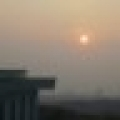# [试题资料] 三年级语文《的、地、得和量词》相关填空，建议收藏看看！ [复制链接]

 01 “的、地、得”的用法口诀 的地得，不一样，用法分别记心上， 左边白，右边勺，名词跟在后面跑。 美丽的花儿绽笑脸，青青的草儿弯下腰， 清清的河水向东流，蓝蓝的天上白云飘， 暖暖的风儿轻轻吹，绿绿的树叶把头摇， 小小的鱼儿水中游，红红的太阳当空照， 左边土，右边也，地字站在动词前， 认真地做操不马虎，专心地上课不大意， 大声地朗读不害羞，从容地走路不着急， 痛快地玩耍来放松，用心地思考解难题， 勤奋地学习要积极，辛勤地劳动花力气， 左边两人就使得，形容词前要用得， 兔子兔子跑得快，乌龟乌龟爬得慢， 青青竹子长得快，参天大树长得慢， 清晨锻炼起得早，加班加点睡得晚， 欢乐时光过得快，考试题目出得难。 名词前面是“白勺” 动词跟着“土也”跑 形动后补“双人”到 默记五遍脑开窍 以上几种用法，可以写成下面几种公式： 修饰限词+“的”+名词 修饰限词+“地”+动词 动词（形容词）+“得”+补充说明 1、地＋动词： 满意地(点头)  快活地(成长)  伤心地(哭泣) 顺利地(打出)  亲切地(问)  骄傲地(说) 兴奋地(唱歌)  飞快地(跑)  甜甜地(笑) 沉着地(操纵)  高兴地(唱、跳)  静静地(想) 仔细地(听、算)  惊奇地(问)  深情地(说、看) 赞许地(点头)  悄悄地(走)  紧紧地(握住) 轻轻地(说)  呆呆地(望)  牢牢地(抓住) 安稳地(睡)  叽叽喳喳地(唱)  摇摇摆摆地(走) (高兴)地说  (沉着)地操纵着  (大声)地回答 (认真)地观看  (顺利)地完成  (紧紧)地拥抱 (开心)地笑了  (大口大口)地吃起来  (奇怪)地问 (满意)地点点头  (仔细)地看  (苦苦) 地等待 (快乐)地跳起舞来  (悄悄)地低语  (快乐)地成长 (尽情)地游戏  (牢牢)地记  (静静)地听 (慢慢)地走 2、的＋名词： 例：可爱的（小猴子） 满意的(笑容)  快活的(孩子)  温柔的(妈妈) 清澈的(河水)  奇怪的(石头)  可爱的(小鸟) 高大的(树木)  健康的(身体)  羡慕的(眼光) 金黄的(谷子)  遥远的(地方)  燃烧的(火把) 红红的(太阳)  漂亮的(书包)  巨大的(石头) 勤劳的(人们)  伤心的(孩子)  伟大的(祖国) 动听的(歌声)  火红的(旗帜)  慈祥的(老人) 难忘的(日子)  寒冷的(冬天)  平静的(心情) 和蔼可亲的(样子)  机灵的(小鸟)  宽阔的(大路) 快乐的(日子)  热烈的(掌声)  出色的(表演) 美丽的(风景)  绿色的(叶子)  蓝蓝的(天空) 安静的(教室)  激动的(心情)  著名的(画家) 雪白的(被子)  细长的(丝瓜)  翠绿的(小草) 特殊的(日子)  清新的(空气)  安静的(小院) 茂盛的(树叶)  长长的(小路)  高高的(山峰) 优美的(舞蹈、动作)  可爱的(小狗) 绿毯似的(草坪)  洁白的(鸽子)  美丽的(花儿) 成功的(实验)  金色的(沙子)  川流不息的(车子) 拼成图案的(花坛)  又宽又长的(马路) (美丽)的图画  (金色)的波浪  (红红)的火把 (火红)的太阳  (呼呼)的北风  (可爱)的人们 (红红)的脸颊  (高高)的山峰  (漂亮)的裙子 (白白)的云海  (美丽)的景色  (巨大)的岩石 (甜甜)的微笑  (快乐)的日子  (亲切)的问候 (无边无际)的海洋  (伤心)的泪水 (干净)的教室  (美丽)的公园  (激动)的心情 (美丽)的花坛  (胖胖)的身体  (美丽)的鲜花 (清新)的空气  (细细)的树枝  (青青)的叶子 (细细)的腰  (红红)的枣子  (漂亮)的衣服 (绿绿)的树苗  (快乐)的时刻  (难闻)的气味 (雪白)的羽毛  (白白)的朝雾  (可爱)的葫芦 (圆圆)的南瓜  (快乐)的日子  (火红)的旗帜 (干净)的校园  (安静)的小院  (明亮)的窗户　 (闪闪)的星星  (红红)的灯笼  (美丽)的城市 (精美)的风筝  (安静)的教室  (长长)的小溪 (金色)的波浪  (红红)的火把  (漂亮)的雄鸡 (坚硬)的铠甲  (绿绿)的枝叶  (可爱)的笑容 (平静)的心情  (丰收)的果实  (雪白)的鸽子 (宽阔)的广场  (绿色)的草坪  (各种各样)的图形 (美丽)的雪景  (白白)的棉花  (细长)的葫芦藤 (白白)的小花  (可爱)的小葫芦  (红红)的枣子 (亮晶晶)的葡萄  (金色)的谷粒  (亲爱)的朋友 (明亮)的眼光  (大大)的眼睛  (美丽)的地方 (好看)的书  (长长)的波纹  (变化)的山峦 (清清)的湖水  (广阔)的树林  (紧张)的时刻 (无边无际)的森林  (红红)的高粱  (开心)的生日 (绿绿)的麦苗  (金色)的稻子  (美丽)的公主 (又大又红)的苹果  (长长)的黄河 3、得+怎么了（事情发展的结果） 乐得(哈哈大笑)  高兴得(跳了起来) 激动得(拍起手来)  兴奋得(大叫) 玩得(满头大汗)  急得(团团转) 伤心得( 流下了眼泪) 觉得(很奇怪) 4、填写量词： 例：一（ 只 ）小鸟 名词：指人或东西、事物的词， 在数量词后面总是名词。 一(架)风车  一(棵)柳树  一(口)水井 一(头)大象  一(片)雪花  一(辆)汽车 一(幅)地图  一(份)文件  一(座)城市 一(面)红旗  一(条)马路  一(块)巨石 一(台)电脑  一(双)眼睛  一(个)广场 一(位)劳动委员  一(头)牛  两(匹)马 三(条)鲤鱼  一(片)树叶  一(枝)神笔 一(件)事情  一(本)图书  一(双)手 一(只)小羊  一(朵)白云  一(个)气球 一(支)粉笔  一(片)树叶  一(把)椅子 一(张)小脸  一(节)课  一(个)园子 一(首)歌曲  一(个)太阳  一(个)游戏 一(条)大鱼  一(棵)大树  一(片)掌声 一(件)衣服  一(匹)骆驼  一(个)委员 一(本)书  一(张)纸  四(只)鸭 五(本)书  六(枝)笔  一(块)围巾 七(棵)果树  八(朵)花  九(架)飞机 一(条)小河  十(辆)车  一(座)山 一(瓶)汽水  一(张)床  一(架)钢琴 一(只)鸡  一(头)毛驴  一(句)话 一(个)脚印  一(把、滴)汗  一(支)歌 一(件)礼物  一(颗)草莓  一(家)商店 一(颗)心  两(朵)花  一(座)高山 一(面)国旗  一(口)气  五(根)柱子 一(座)山峰  一( 块)石头  一(面)镜子 一(条)波纹  一(位)仙人  一(只)雄鹰 一(阵)秋风  四(把)扇子  一(个)月亮 一(串)葡萄  一(位)老师  三(条)绳子 一(把)椅子  一(张)桌子  一(条)被子 一(口)井  一(台)电脑  一(把)二胡 一支(歌)  一只(鸟)  一枝(笔) 一盘(菜)  一块(石头) 一把(椅子) 一本(书) 一架(飞机)  一个(人) 一片(云)  一头(牛)  一双(手) 一座(山)  一盒(笔)  一窝(小鸟) 一袋(米)  一棵(树)  一匹(马) 一条(河)  一阵(风)  一串(珠子) 一位(老师)  一道(彩虹)  一次(作业) 一台(电视)  一则(日记)  一枝(笔) 一片片(叶子)  一把把(小刀)  一条条(河) 一盆盆(水)  一个个(苹果)  一包包(米) 一块块(石头)  一只只(小鸟)  一枝枝(笔) 一棵棵(树)  一串串(珠子)  一群群(羊) 一面面(旗帜)  一座座(山)  一张张(脸) 一句句(话)  一本本(书)  一盏盏(灯) 一双双(手)  一台台(电脑)  一层层(房子) 一排排(座位)  一件件(衣服)  一首首(歌)

2019-8-7 10:11 显示全部楼层

 感谢分享点赞您需要登录后才可以回帖 登录 | 注册使用家长帮，赚更多元宝！ 回帖后跳转到最后一页

•••